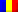epxn3gmmeegy384y9epbyqncqy509l

Anoucka, a Romanian luxury brand seen in Vogue, Elle and Forbes!

22.00 USD

(1 customer review)

A lovely deep ivory hairband for girls. Lightly padded, the flexible band is covered in a soft and lightweight linen and cotton blend fabric, with  beautiful textured ruffles and pearls on the edge.

Recommended size – 12 Months +

Category:

## ``` 1 review for Caroline Headband```

1. ``

``` Cristina Firent – July 3, 2023```

`Vreau doar sa spun ca pozele produselor nu justifica cat de frumoase sunt produsele Anoucka in realitate. Calitate premium, detalii atent lucrate. Ramanem clienti fideli`

`Show only reviews in English (0)`

``` {"@context":"https:\/\/schema.org\/","@graph":[{"@context":"https:\/\/schema.org\/","@type":"Product","@id":"https:\/\/www.anoucka.com\/product\/caroline-headband-for-girls\/#product","name":"Caroline Headband","url":"https:\/\/www.anoucka.com\/product\/caroline-headband-for-girls\/","description":"A lovely deep ivory hairband for girls. Lightly padded, the flexible band is covered in a soft and lightweight linen and cotton blend fabric, with\u00a0 beautiful textured ruffles and pearls on the edge.\r\n\r\nRecommended size - 12 Months +","image":"https:\/\/www.anoucka.com\/wp-content\/uploads\/2023\/03\/1200px-23-03-29-0090.jpg","sku":251878,"offers":[{"@type":"Offer","price":"92.36","priceValidUntil":"2024-12-31","priceSpecification":{"price":"92.36","priceCurrency":"RON","valueAddedTaxIncluded":"true"},"priceCurrency":"RON","availability":"http:\/\/schema.org\/InStock","url":"https:\/\/www.anoucka.com\/product\/caroline-headband-for-girls\/","seller":{"@type":"Organization","name":"Anoucka","url":"https:\/\/www.anoucka.com"}}],"aggregateRating":{"@type":"AggregateRating","ratingValue":"5.00","reviewCount":1},"review":[{"@type":"Review","reviewRating":{"@type":"Rating","bestRating":"5","ratingValue":"5","worstRating":"1"},"author":{"@type":"Person","name":"Cristina Firent"},"reviewBody":"Vreau doar sa spun ca pozele produselor nu justifica cat de frumoase sunt produsele Anoucka in realitate. Calitate premium, detalii atent lucrate. Ramanem clienti fideli","datePublished":"2023-07-03T09:06:46+03:00"}]},{"@context":"https:\/\/schema.org\/","@type":"BreadcrumbList","itemListElement":[{"@type":"ListItem","position":1,"item":{"name":"Home","@id":"https:\/\/www.anoucka.com"}},{"@type":"ListItem","position":2,"item":{"name":"HEADBANDS","@id":"https:\/\/www.anoucka.com\/product-category\/headbands\/"}},{"@type":"ListItem","position":3,"item":{"name":"Caroline Headband","@id":"https:\/\/www.anoucka.com\/product\/caroline-headband-for-girls\/"}}]}]} (function() { var file = ["https:\/\/www.anoucka.com\/wp-content\/et-cache\/251878\/et-divi-dynamic-tb-231469-tb-231384-251878-late.css"]; var handle = document.getElementById('divi-style-parent-inline-inline-css'); var location = handle.parentNode; if (0===document.querySelectorAll('link[href="' + file + '"]').length) { var link = document.createElement('link'); link.rel = 'stylesheet'; link.id = 'et-dynamic-late-css'; link.href = file; location.insertBefore(link, handle.nextSibling); } })();!function(t,e){"object"==typeof exports&&"undefined"!=typeof module?module.exports=e():"function"==typeof define&&define.amd?define(e):(t="undefined"!=typeof globalThis?globalThis:t||self).LazyLoad=e()}(this,function(){"use strict";function e(){return(e=Object.assign||function(t){for(var e=1;e<arguments.length;e++){var n,a=arguments[e];for(n in a)Object.prototype.hasOwnProperty.call(a,n)&&(t[n]=a[n])}return t}).apply(this,arguments)}function i(t){return e({},it,t)}function o(t,e){var n,a="LazyLoad::Initialized",i=new t(e);try{n=new CustomEvent(a,{detail:{instance:i}})}catch(t){(n=document.createEvent("CustomEvent")).initCustomEvent(a,!1,!1,{instance:i})}window.dispatchEvent(n)}function l(t,e){return t.getAttribute(gt+e)}function c(t){return l(t,bt)}function s(t,e){return function(t,e,n){e=gt+e;null!==n?t.setAttribute(e,n):t.removeAttribute(e)}(t,bt,e)}function r(t){return s(t,null),0}function u(t){return null===c(t)}function d(t){return c(t)===vt}function f(t,e,n,a){t&&(void 0===a?void 0===n?t(e):t(e,n):t(e,n,a))}function _(t,e){nt?t.classList.add(e):t.className+=(t.className?" ":"")+e}function v(t,e){nt?t.classList.remove(e):t.className=t.className.replace(new RegExp("(^|\\s+)"+e+"(\\s+|\$)")," ").replace(/^\s+/,"").replace(/\s+\$/,"")}function g(t){return t.llTempImage}function b(t,e){!e||(e=e._observer)&&e.unobserve(t)}function p(t,e){t&&(t.loadingCount+=e)}function h(t,e){t&&(t.toLoadCount=e)}function n(t){for(var e,n=[],a=0;e=t.children[a];a+=1)"SOURCE"===e.tagName&&n.push(e);return n}function m(t,e){(t=t.parentNode)&&"PICTURE"===t.tagName&&n(t).forEach(e)}function a(t,e){n(t).forEach(e)}function E(t){return!!t[st]}function I(t){return t[st]}function y(t){return delete t[st]}function A(e,t){var n;E(e)||(n={},t.forEach(function(t){n[t]=e.getAttribute(t)}),e[st]=n)}function k(a,t){var i;E(a)&&(i=I(a),t.forEach(function(t){var e,n;e=a,(t=i[n=t])?e.setAttribute(n,t):e.removeAttribute(n)}))}function L(t,e,n){_(t,e.class_loading),s(t,ut),n&&(p(n,1),f(e.callback_loading,t,n))}function w(t,e,n){n&&t.setAttribute(e,n)}function x(t,e){w(t,ct,l(t,e.data_sizes)),w(t,rt,l(t,e.data_srcset)),w(t,ot,l(t,e.data_src))}function O(t,e,n){var a=l(t,e.data_bg_multi),i=l(t,e.data_bg_multi_hidpi);(a=at&&i?i:a)&&(t.style.backgroundImage=a,n=n,_(t=t,(e=e).class_applied),s(t,ft),n&&(e.unobserve_completed&&b(t,e),f(e.callback_applied,t,n)))}function N(t,e){!e||0<e.loadingCount||0<e.toLoadCount||f(t.callback_finish,e)}function C(t,e,n){t.addEventListener(e,n),t.llEvLisnrs[e]=n}function M(t){return!!t.llEvLisnrs}function z(t){if(M(t)){var e,n,a=t.llEvLisnrs;for(e in a){var i=a[e];n=e,i=i,t.removeEventListener(n,i)}delete t.llEvLisnrs}}function R(t,e,n){var a;delete t.llTempImage,p(n,-1),(a=n)&&--a.toLoadCount,v(t,e.class_loading),e.unobserve_completed&&b(t,n)}function T(o,r,c){var l=g(o)||o;M(l)||function(t,e,n){M(t)||(t.llEvLisnrs={});var a="VIDEO"===t.tagName?"loadeddata":"load";C(t,a,e),C(t,"error",n)}(l,function(t){var e,n,a,i;n=r,a=c,i=d(e=o),R(e,n,a),_(e,n.class_loaded),s(e,dt),f(n.callback_loaded,e,a),i||N(n,a),z(l)},function(t){var e,n,a,i;n=r,a=c,i=d(e=o),R(e,n,a),_(e,n.class_error),s(e,_t),f(n.callback_error,e,a),i||N(n,a),z(l)})}function G(t,e,n){var a,i,o,r,c;t.llTempImage=document.createElement("IMG"),T(t,e,n),E(c=t)||(c[st]={backgroundImage:c.style.backgroundImage}),o=n,r=l(a=t,(i=e).data_bg),c=l(a,i.data_bg_hidpi),(r=at&&c?c:r)&&(a.style.backgroundImage='url("'.concat(r,'")'),g(a).setAttribute(ot,r),L(a,i,o)),O(t,e,n)}function D(t,e,n){var a;T(t,e,n),a=e,e=n,(t=It[(n=t).tagName])&&(t(n,a),L(n,a,e))}function V(t,e,n){var a;a=t,(-1<yt.indexOf(a.tagName)?D:G)(t,e,n)}function F(t,e,n){var a;t.setAttribute("loading","lazy"),T(t,e,n),a=e,(e=It[(n=t).tagName])&&e(n,a),s(t,vt)}function j(t){t.removeAttribute(ot),t.removeAttribute(rt),t.removeAttribute(ct)}function P(t){m(t,function(t){k(t,Et)}),k(t,Et)}function S(t){var e;(e=At[t.tagName])?e(t):E(e=t)&&(t=I(e),e.style.backgroundImage=t.backgroundImage)}function U(t,e){var n;S(t),n=e,u(e=t)||d(e)||(v(e,n.class_entered),v(e,n.class_exited),v(e,n.class_applied),v(e,n.class_loading),v(e,n.class_loaded),v(e,n.class_error)),r(t),y(t)}function \$(t,e,n,a){var i;n.cancel_on_exit&&(c(t)!==ut||"IMG"===t.tagName&&(z(t),m(i=t,function(t){j(t)}),j(i),P(t),v(t,n.class_loading),p(a,-1),r(t),f(n.callback_cancel,t,e,a)))}function q(t,e,n,a){var i,o,r=(o=t,0<=pt.indexOf(c(o)));s(t,"entered"),_(t,n.class_entered),v(t,n.class_exited),i=t,o=a,n.unobserve_entered&&b(i,o),f(n.callback_enter,t,e,a),r||V(t,n,a)}function H(t){return t.use_native&&"loading"in HTMLImageElement.prototype}function B(t,i,o){t.forEach(function(t){return(a=t).isIntersecting||0<a.intersectionRatio?q(t.target,t,i,o):(e=t.target,n=t,a=i,t=o,void(u(e)||(_(e,a.class_exited),\$(e,n,a,t),f(a.callback_exit,e,n,t))));var e,n,a})}function J(e,n){var t;et&&!H(e)&&(n._observer=new IntersectionObserver(function(t){B(t,e,n)},{root:(t=e).container===document?null:t.container,rootMargin:t.thresholds||t.threshold+"px"}))}function K(t){return Array.prototype.slice.call(t)}function Q(t){return t.container.querySelectorAll(t.elements_selector)}function W(t){return c(t)===_t}function X(t,e){return e=t||Q(e),K(e).filter(u)}function Y(e,t){var n;(n=Q(e),K(n).filter(W)).forEach(function(t){v(t,e.class_error),r(t)}),t.update()}function t(t,e){var n,a,t=i(t);this._settings=t,this.loadingCount=0,J(t,this),n=t,a=this,Z&&window.addEventListener("online",function(){Y(n,a)}),this.update(e)}var Z="undefined"!=typeof window,tt=Z&&!("onscroll"in window)||"undefined"!=typeof navigator&&/(gle|ing|ro)bot|crawl|spider/i.test(navigator.userAgent),et=Z&&"IntersectionObserver"in window,nt=Z&&"classList"in document.createElement("p"),at=Z&&1<window.devicePixelRatio,it={elements_selector:".lazy",container:tt||Z?document:null,threshold:300,thresholds:null,data_src:"src",data_srcset:"srcset",data_sizes:"sizes",data_bg:"bg",data_bg_hidpi:"bg-hidpi",data_bg_multi:"bg-multi",data_bg_multi_hidpi:"bg-multi-hidpi",data_poster:"poster",class_applied:"applied",class_loading:"litespeed-loading",class_loaded:"litespeed-loaded",class_error:"error",class_entered:"entered",class_exited:"exited",unobserve_completed:!0,unobserve_entered:!1,cancel_on_exit:!0,callback_enter:null,callback_exit:null,callback_applied:null,callback_loading:null,callback_loaded:null,callback_error:null,callback_finish:null,callback_cancel:null,use_native:!1},ot="src",rt="srcset",ct="sizes",lt="poster",st="llOriginalAttrs",ut="loading",dt="loaded",ft="applied",_t="error",vt="native",gt="data-",bt="ll-status",pt=[ut,dt,ft,_t],ht=[ot],mt=[ot,lt],Et=[ot,rt,ct],It={IMG:function(t,e){m(t,function(t){A(t,Et),x(t,e)}),A(t,Et),x(t,e)},IFRAME:function(t,e){A(t,ht),w(t,ot,l(t,e.data_src))},VIDEO:function(t,e){a(t,function(t){A(t,ht),w(t,ot,l(t,e.data_src))}),A(t,mt),w(t,lt,l(t,e.data_poster)),w(t,ot,l(t,e.data_src)),t.load()}},yt=["IMG","IFRAME","VIDEO"],At={IMG:P,IFRAME:function(t){k(t,ht)},VIDEO:function(t){a(t,function(t){k(t,ht)}),k(t,mt),t.load()}},kt=["IMG","IFRAME","VIDEO"];return t.prototype={update:function(t){var e,n,a,i=this._settings,o=X(t,i);{if(h(this,o.length),!tt&&et)return H(i)?(e=i,n=this,o.forEach(function(t){-1!==kt.indexOf(t.tagName)&&F(t,e,n)}),void h(n,0)):(t=this._observer,i=o,t.disconnect(),a=t,void i.forEach(function(t){a.observe(t)}));this.loadAll(o)}},destroy:function(){this._observer&&this._observer.disconnect(),Q(this._settings).forEach(function(t){y(t)}),delete this._observer,delete this._settings,delete this.loadingCount,delete this.toLoadCount},loadAll:function(t){var e=this,n=this._settings;X(t,n).forEach(function(t){b(t,e),V(t,n,e)})},restoreAll:function(){var e=this._settings;Q(e).forEach(function(t){U(t,e)})}},t.load=function(t,e){e=i(e);V(t,e)},t.resetStatus=function(t){r(t)},Z&&function(t,e){if(e)if(e.length)for(var n,a=0;n=e[a];a+=1)o(t,n);else o(t,e)}(t,window.lazyLoadOptions),t});!function(e,t){"use strict";function a(){t.body.classList.add("litespeed_lazyloaded")}function n(){console.log("[LiteSpeed] Start Lazy Load Images"),d=new LazyLoad({elements_selector:"[data-lazyloaded]",callback_finish:a}),o=function(){d.update()},e.MutationObserver&&new MutationObserver(o).observe(t.documentElement,{childList:!0,subtree:!0,attributes:!0})}var d,o;e.addEventListener?e.addEventListener("load",n,!1):e.attachEvent("onload",n)}(window,document); ```